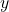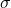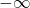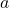## Numerical stability of binary cross entropy loss and the log-sum-exp trickWhen training a binary classifier, cross entropy (CE) loss is usually used as squared error loss cannot distinguish bad predictions from extremely bad predictions. The CE loss is defined as follows:whereis the probability of the sample falling in the positive class., whereis a sigmoid function.

When implementing CE loss, we could calculatefirst and then plugin the definition of CE loss. However, there is a problem with this in practice. At the beginning of training, a positive example might be confidently classified as a negative example, implying. Ifis small enough, it could be smaller than the smallest floating point value i.e. numerically zero. Then we getif we take the log of 0 when computing the cross-entropy. To tackle this potential numerical stability issue, the logistic function and cross-entropy are usually combined into one in package in Tensorflow and PytorchStill, the numerical stability issue is not completely under control sincecould blow up if z is a large negative number. To tackle this potential problem, the “log-sum-exp” trick is used to shift the center of the exponential sum. The log-sum-exp trick is described as followsUsing this formula, we can force the greatest value to be zero even if other values would underflow. Socan bein practice.

References:
•